# How to sum in a For or a While Loop in PythonLast updated: Feb 19, 2023
5 min## #Sum in a for loop in Python

To sum in a for loop in Python:

1. Declare a new variable and set it to `0`.
2. Use a `for` loop to iterate over a sequence of numbers.
3. Reassign the variable to its value plus the current number.
main.py
```Copied!```my_list = [2, 4, 6, 8]

total = 0

for num in my_list:
total += num

print(total)  # 👉️ 20
``````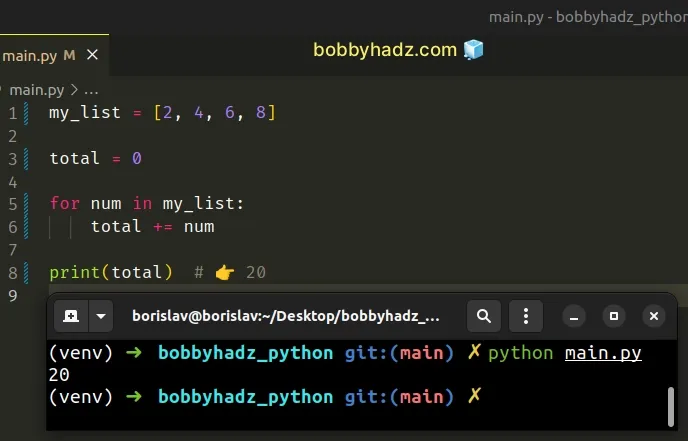We used a for loop to sum the numbers in a list.

The first step is to declare a new variable and initialize it to `0`.

On each iteration, we use the `+=` operator to reassign the variable to its current value plus the current number.

The following 2 lines of code achieve the same result:

• `total += num`
• `total = total + num`

Here is an example that uses the longer reassignment syntax.

main.py
```Copied!```my_list = [2, 4, 6, 8]

total = 0

for num in my_list:
total = total + num

print(total)  # 👉️ 20
``````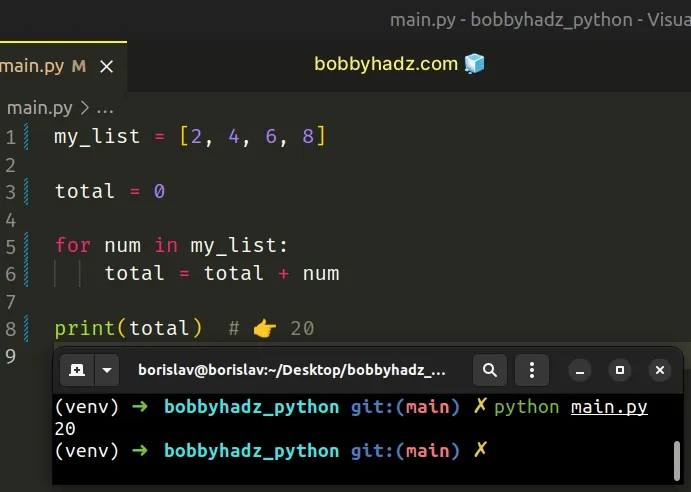## #Sum the numbers in a certain range using a `for` loop

If you need to add the numbers in a certain range using a `for` loop, create the range with the `range()` class.

main.py
```Copied!```total = 0

for num in range(1, 5):
total += num

print(total)  # 👉️ 10

print(list(range(1, 5)))  # 👉️ [1, 2, 3, 4]
``````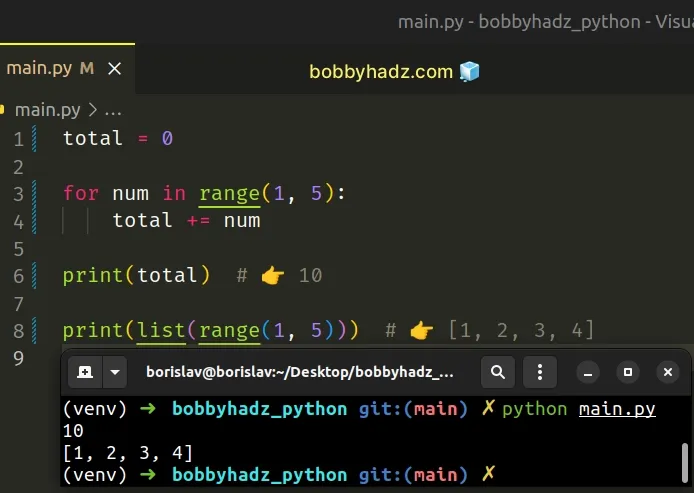The range class is commonly used for looping a specific number of times in `for` loops and takes the following parameters:

NameDescription
`start`An integer representing the start of the range (defaults to `0`)
`stop`Go up to, but not including the provided integer
`step`Range will consist of every N numbers from `start` to `stop` (defaults to `1`)

## #Sum numbers taken from user input in a for loop

If you need to sum numbers taken from user input in a for loop, use the `input()` function.

main.py
```Copied!```# 👇️ user enters 1 2 3 4
user_input = input('Enter space-separated numbers: ')

my_list = list(map(int, user_input.split()))

print(my_list)  # 👉️ [1, 2, 3, 4]

total = 0

for num in my_list:
total += num

print(total)  # 👉️ 10
``````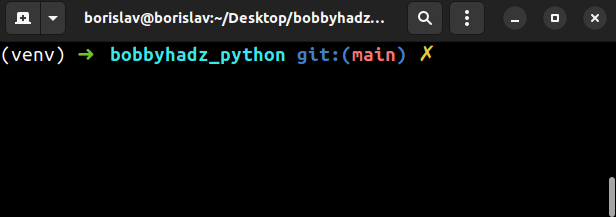The input function takes an optional `prompt` argument and writes it to standard output without a trailing newline.

The `input()` function is guaranteed to return a string even if the user enters a number.

We used the `str.split()` function to split the string on each space.

The str.split() method splits the string into a list of substrings using a delimiter.

The method takes the following 2 parameters:

NameDescription
separatorSplit the string into substrings on each occurrence of the separator
maxsplitAt most `maxsplit` splits are done (optional)

If the separator is not found in the string, a list containing only 1 element is returned.

We used a whitespace separator in the example, but you use any other separator that suits your use case.

Here is an example that splits the user-provided string on each comma.

main.py
```Copied!```# 👇️ user enters 1,2,3,4
user_input = input('Enter comma-separated numbers: ')

my_list = list(map(int, user_input.split(',')))

print(my_list)  # 👉️ [1, 2, 3, 4]

total = 0

for num in my_list:
total += num

print(total)  # 👉️ 10
``````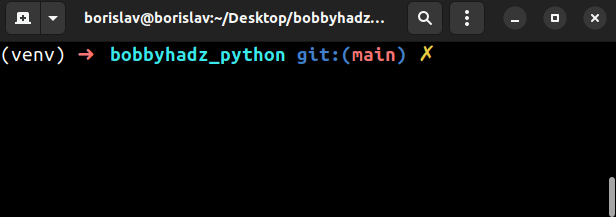After splitting the string, we get a list of strings, so we used the `map()` function to convert each string in the list to an integer.

main.py
```Copied!```# 👇️ user enters 1,2,3,4
user_input = input('Enter comma-separated numbers:')

# 👇️ ['1', '2', '3', '4']
print(user_input.split(','))
``````

The map() function takes a function and an iterable as arguments and calls the function with each item of the iterable.

The `map()` function passes each string to the int() class and converts it to an integer.

## #Sum of N numbers using a while loop in Python

To get the sum of N numbers using a while loop:

1. Iterate for as long as the number is greater than `0`.
2. On each iteration, decrement the number by `1`.
3. On each iteration, increment the total by the number.
main.py
```Copied!```sum_of_numbers = 0

num = 5

while num > 0:
# 👇️ reassign sum to sum + num
sum_of_numbers += num

# 👇️ reassign num to num - 1
num -= 1

print(sum_of_numbers)  # 👉️ 15  (5 + 4 + 3 + 2 + 1)

print(num)  # 👉️ 0
``````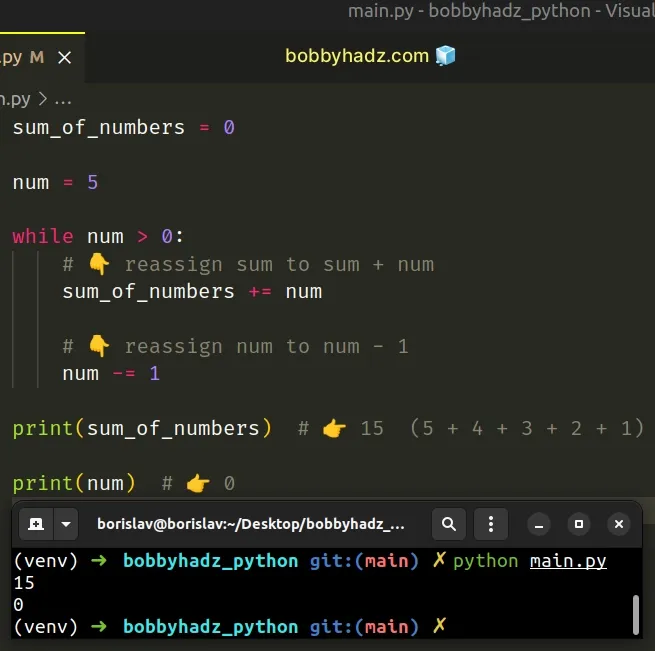The `while` loop in the example iterates for as long as the `num` variable stores a value greater than `0`.

On each iteration, we use the `+=` operator to reassign the `sum_of_numbers` variable to its current value plus `num`.

To move toward the base case, we also use the `-=` operator to reassign the `num` variable to its value minus `1`.

The following 2 lines of code achieve the same result:

• `sum_of_numbers += num`
• `sum_of_numbers = sum_of_numbers + num`

Similarly, the `-=` operator is also a shorthand:

• `num -= 1`
• `num = num - 1`
Make sure to specify a base case that has to be met to exit the `while` loop, otherwise you might end up with an infinite loop.

## #Sum of N numbers using a while True loop

A commonly used alternative is to use a `while True` loop with a `break` statement.

main.py
```Copied!```sum_of_numbers = 0

num = 5

while True:
# 👇️ reassign sum to sum + num
sum_of_numbers += num

# 👇️ reassign num to num - 1
num -= 1

# 👇️ if num is equal to or less than `0`, break out of loop
if num <= 0:
break

print(sum_of_numbers)  # 👉️ 15  (5 + 4 + 3 + 2 + 1)

print(num)  # 👉️ 0
``````

The `while True` loop iterates until it is interrupted by a `break` statement.

I've also written an article on how to sum all values in a dictionary or a list of dictionaries.